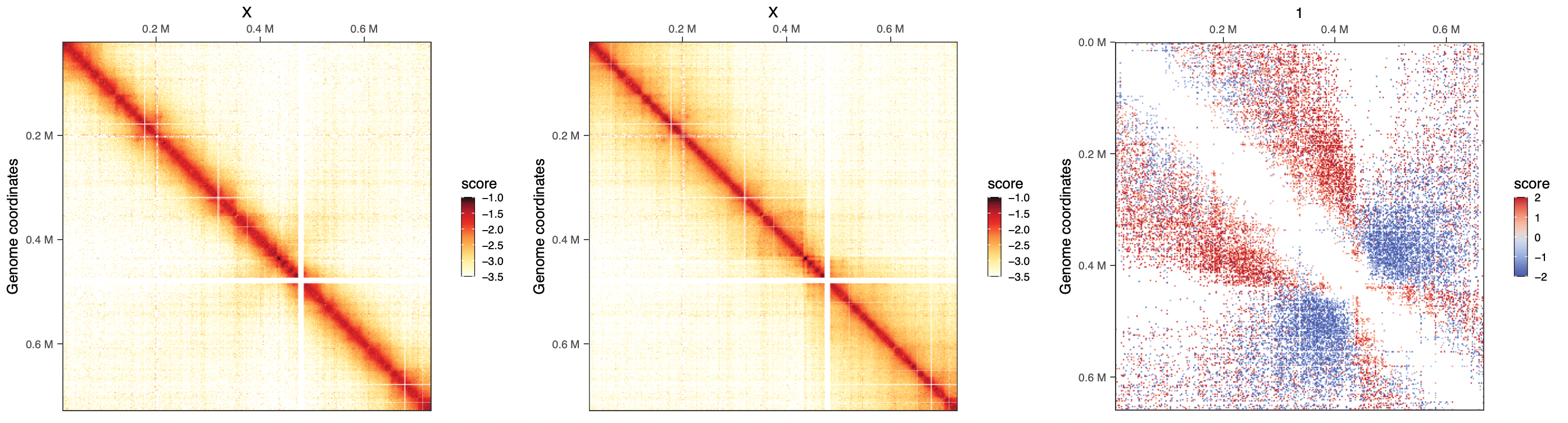# Workflow 1: Distance-dependent interactions across yeast mutants

Aims

This chapter illustrates how to:

• Compute P(s) of several samples and compare them
• Compute distance-adjusted correlation between Hi-C datasets with `HiCRep`
• Perform differential interaction analysis between Hi-C datasets with `multiHiCcompare`
Datasets

We leverage seven yeast datasets in this notebook. They are all available from SRA:

• `SRR8769554`: WT yeast strain, G1 phase (rep1)
• `SRR10687276`: WT yeast strain, G1 phase (rep12)
• `SRR8769549`: WT yeast strain, G2/M phase (rep1)
• `SRR10687281`: WT yeast strain, G2/M phase (rep12)
• `SRR8769551`: wpl1 mutant yeast strain, G2/M phase (rep1)
• `SRR10687278`: wpl1 mutant yeast strain, G2/M phase (rep2)
• `SRR8769555`: wpl1/eco1 mutant yeast strain, G2/M phase

## Recovering data from SRA

The easiest for this is to directly fetch files from SRA from their FTP server. We can do so using the base `download.file` function.

Note

The next two code chunks illustrate how to do download and process Hi-C reads from SRA, but they are not actually executed when rendering this website as it would take a significant amount of time.

``````# !! This code is not actually executed !!
dir.create('data')

We will map each pair of fastqs on the yeast genome reference (`R64-1-1`) using `HiCool`.

``````# !! This code is not actually executed !!
library(HiCool)
samples <- c(
'WT_G1_rep1',
'WT_G1_rep2',
'WT_G2M_rep1',
'WT_G2M_rep2',
'wpl1_G2M_rep1',
'wpl1_G2M_rep2',
'wpl1eco1_G2M'
)
purrr::map(samples, ~ HiCool(
r1 = paste0('data/', .x, '_R1.fastq.gz'),
r2 = paste0('data/', .x, '_R2.fastq.gz'),
genome = 'R64-1-1',
restriction = 'DpnII',
iterative = FALSE,
output = 'data/HiCool/',
scratch = '/data/scratch/'
))``````

Processed samples are put in `data/HiCool` directory. `CoolFile` objects are pointers to individual contact matrices. We can create such objects by using the `importHiCoolFolder` utility function.

``````cfs <- list(
WT_G1_rep1 = importHiCoolFolder('data/HiCool', 'GK8ISZ'),
WT_G1_rep2 = importHiCoolFolder('data/HiCool', 'SWZTO0'),
WT_G2M_rep1 = importHiCoolFolder('data/HiCool', '3KHHUE'),
WT_G2M_rep2 = importHiCoolFolder('data/HiCool', 'UVNG7M'),
wpl1_G2M_rep1 = importHiCoolFolder('data/HiCool', 'Q4KX6Z'),
wpl1_G2M_rep2 = importHiCoolFolder('data/HiCool', '3N0L25'),
wpl1eco1_G2M = importHiCoolFolder('data/HiCool', 'LHMXWE')
)
cfs``````

Now that these pointers have been defined, Hi-C contact matrices can be seamlessly imported in R with `import`.

``````library(purrr)
library(HiCExperiment)
hics <- map(cfs, import)
hics
## \$WT_G1_rep1
## `HiCExperiment` object with 5,454,145 contacts over 12,079 regions
## -------
## fileName: "../OHCA-data/HiCool/matrices/W303_G1_WT_rep1^mapped-S288c^GK8ISZ.mcool"
## focus: "whole genome"
## resolutions(5): 1000 2000 4000 8000 16000
## active resolution: 1000
## interactions: 3347524
## scores(2): count balanced
## topologicalFeatures: compartments(0) borders(0) loops(0) viewpoints(0)
## pairsFile: ../OHCA-data/HiCool/pairs/W303_G1_WT_rep1^mapped-S288c^GK8ISZ.pairs
##
## \$WT_G1_rep2
## `HiCExperiment` object with 12,068,214 contacts over 12,079 regions
## -------
## fileName: "../OHCA-data/HiCool/matrices/W303_G1_WT_rep2^mapped-S288c^SWZTO0.mcool"
## focus: "whole genome"
## resolutions(5): 1000 2000 4000 8000 16000
## active resolution: 1000
## interactions: 6756099
## scores(2): count balanced
## topologicalFeatures: compartments(0) borders(0) loops(0) viewpoints(0)
## pairsFile: ../OHCA-data/HiCool/pairs/W303_G1_WT_rep2^mapped-S288c^SWZTO0.pairs
##
## ... ``````

## Plotting chromosome-wide matrices of merged replicates

We can merge replicates with the `merge` function, and `map` the `plotMatrix` function over the resulting list of `HiCExperiment`s.

``````library(HiContacts)
chr <- 'X'
merged_replicates <- list(
WT_G1 = merge(hics[][chr], hics[][chr]),
WT_G2M = merge(hics[][chr], hics[][chr]),
wpl1_G2M = merge(hics[][chr], hics[][chr]),
wpl1eco1_G2M = hics[][chr]
)
library(dplyr)
library(ggplot2)
maps <- imap(merged_replicates, ~ plotMatrix(
.x, use.scores = 'balanced', limits = c(-3.5, -1.5), caption = FALSE
) + ggtitle(.y))
cowplot::plot_grid(plotlist = maps, nrow = 1)``````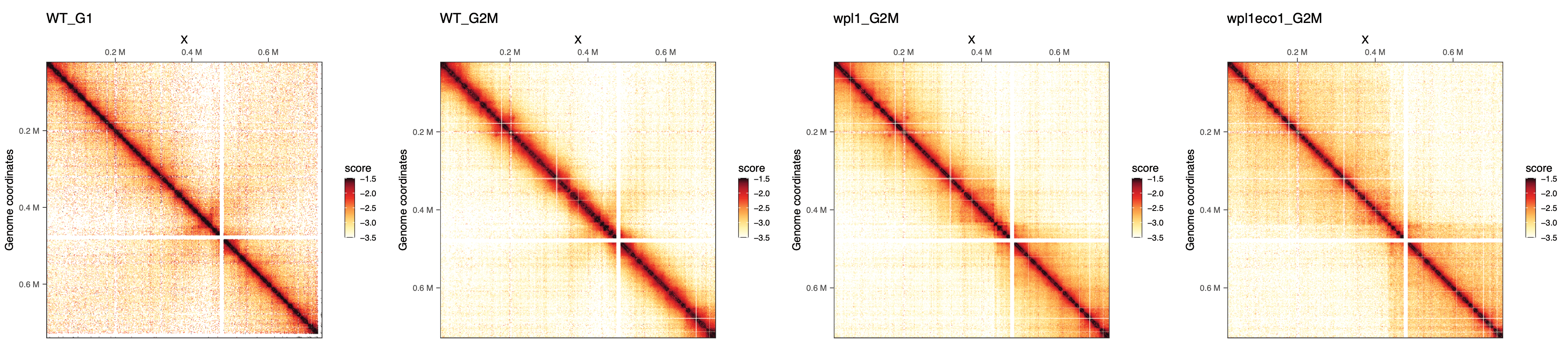We can already note that long-range contacts seem to increase in frequency, in G2/M vs G1, in wpl1 vs WT and in wpl1/eco1 vs wpl1.

## Compute P(s) per replicate and plot it

Still using the `map` function, we can compute average P(s) for each replicate.
Computation of the P(s) will take some time, as millions of pairs have to be imported in memory, but it will be accurate at the base resolution, rather than bin resolution from matrices.

Note

Since matrices were imported after `HiCool` processing with the `importHiCoolFolder`, the associated `.pairs` file has been automatically added to each `HiCExperiment` object!

The computed P(s) is stored for each sample as a `tibble`.

``````pairsFile(hics[])
ps <- imap(hics, ~ distanceLaw(.x) |> mutate(sample = .y))
## Importing pairs file ../OHCA-data/HiCool/pairs/W303_G1_WT_rep1^mapped-S288c^GK8ISZ.pairs in memory. This may take a while...
## |===============================================================| 100% 318 MB
## Importing pairs file ../OHCA-data/HiCool/pairs/W303_G1_WT_rep2^mapped-S288c^SWZTO0.pairs in memory. This may take a while...
## |===============================================================| 100% 674 MB
## Importing pairs file ../OHCA-data/HiCool/pairs/W303_G2M_WT_rep1^mapped-S288c^3KHHUE.pairs in memory. This may take a while...
## |===============================================================| 100% 709 MB
## Importing pairs file ../OHCA-data/HiCool/pairs/W303_G2M_WT_rep2^mapped-S288c^UVNG7M.pairs in memory. This may take a while...
## |==============================================================| 100% 1683 MB
## Importing pairs file ../OHCA-data/HiCool/pairs/W303_G2M_wpl1_rep1^mapped-S288c^Q4KX6Z.pairs in memory. This may take a while...
## |==============================================================| 100% 1269 MB
## Importing pairs file ../OHCA-data/HiCool/pairs/W303_G2M_wpl1_rep2^mapped-S288c^3N0L25.pairs in memory. This may take a while...
## |==============================================================| 100% 1529 MB
## Importing pairs file ../OHCA-data/HiCool/pairs/W303_G2M_wpl1-eco1^mapped-S288c^LHMXWE.pairs in memory. This may take a while...
## |==============================================================| 100% 1036 MB
ps[]
## # A tibble: 133 x 6
##   binned_distance          p     norm_p norm_p_unity slope sample
##             <dbl>      <dbl>      <dbl>        <dbl> <dbl> <chr>
## 1               1 0.000154   0.000154         249.   0     WT_G1_rep1
## 2               2 0.0000563  0.0000563         91.2  0.702 WT_G1_rep1
## 3               3 0.0000417  0.0000417         67.5  0.699 WT_G1_rep1
## 4               4 0.00000835 0.00000835        13.5  0.696 WT_G1_rep1
## 5               5 0.00000501 0.00000501         8.10 0.693 WT_G1_rep1
## 6               6 0.00000250 0.00000250         4.05 0.690 WT_G1_rep1
## # ... with 127 more rows``````

We can bind all `tibbles` together and plot P(s) and their slope for each sample.

``````df <- list_rbind(ps)
plotPs(
df, aes(x = binned_distance, y = norm_p,
group = sample, color = sample)
)
plotPsSlope(
df, aes(x = binned_distance, y = slope,
group = sample, color = sample)
)``````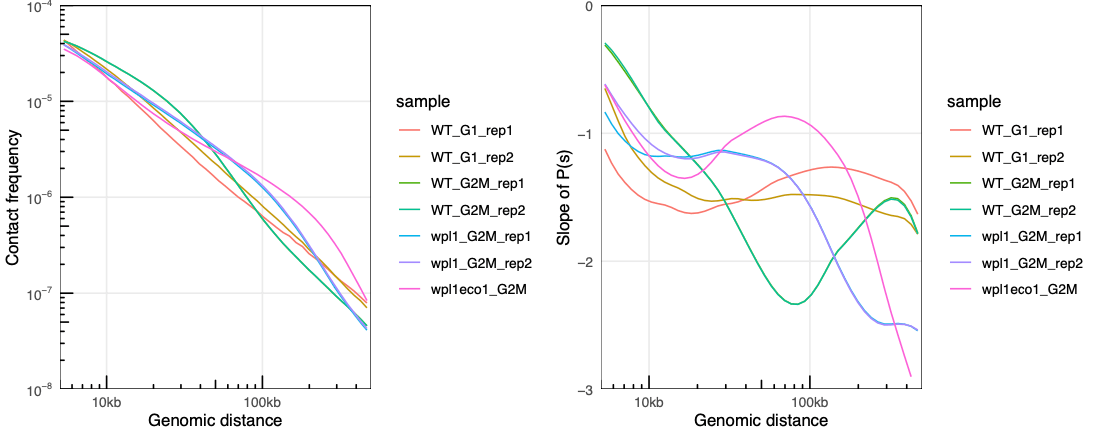## Correlation between replicates with `hicrep`

`hicrep` is a popular package to compute stratum-adjusted correlations between Hi-C datasets. “Stratum” refers to the distance from the main diagonal: with increase distance from the main diagonal, interactions of the DNA polymer are bound to decrease. `hicrep` computes a “per-stratum” correlation score and computes a weighted average correlation for entire chromosomes.

We can check the documentation for `hicrep` main function, `get.scc`. This tells us that `mat1` and `mat2` `n*n` intrachromosomal contact maps of raw `counts` should be provided. Fortunately, `HiCExperiment` objects can easily be coerced into actual dense matrices using `as.matrix()` function.

Important

Make sure to use the `count` scores, which are required by `hicrep`.

We can calculate the overall stratum-corrected correlation score over the chromosome `IV` between the two `G2M` WT replicates.

``````library(hicrep)
scc <- get.scc(
hics[['WT_G2M_rep1']]["IV"] |> as.matrix(use.scores = 'count'),
hics[['WT_G2M_rep2']]["IV"] |> as.matrix(use.scores = 'count'),
resol = 1000, h = 2, lbr = 5000, ubr = 50000
)
names(scc)
##  "corr" "wei"  "scc"  "std"
scc\$scc
##           [,1]
## [1,] 0.9785691``````

This can be generalized to all pairwise combinations of Hi-C datasets.

``````library(purrr)
library(dplyr)
library(ggplot2)
mats <- map(hics, ~ .x["IV"] |> as.matrix(use.scores = 'count'))
df <- map(1:7, function(i) {
map(1:7, function(j) {
data.frame(
i = names(hics)[i],
j = names(hics)[j],
scc = hicrep::get.scc(mats[[i]], mats[[j]], resol = 1000, h = 2, lbr = 5000, ubr = 200000)\$scc
) |>
mutate(i = factor(i, names(cfs))) |>
mutate(j = factor(j, names(cfs)))
}) |> list_rbind()
}) |> list_rbind()
ggplot(df, aes(x = i, y = j, fill = scc)) +
geom_tile() +
scale_x_discrete(guide = guide_axis(angle = 90)) +
theme_bw() +
coord_fixed(ratio = 1) +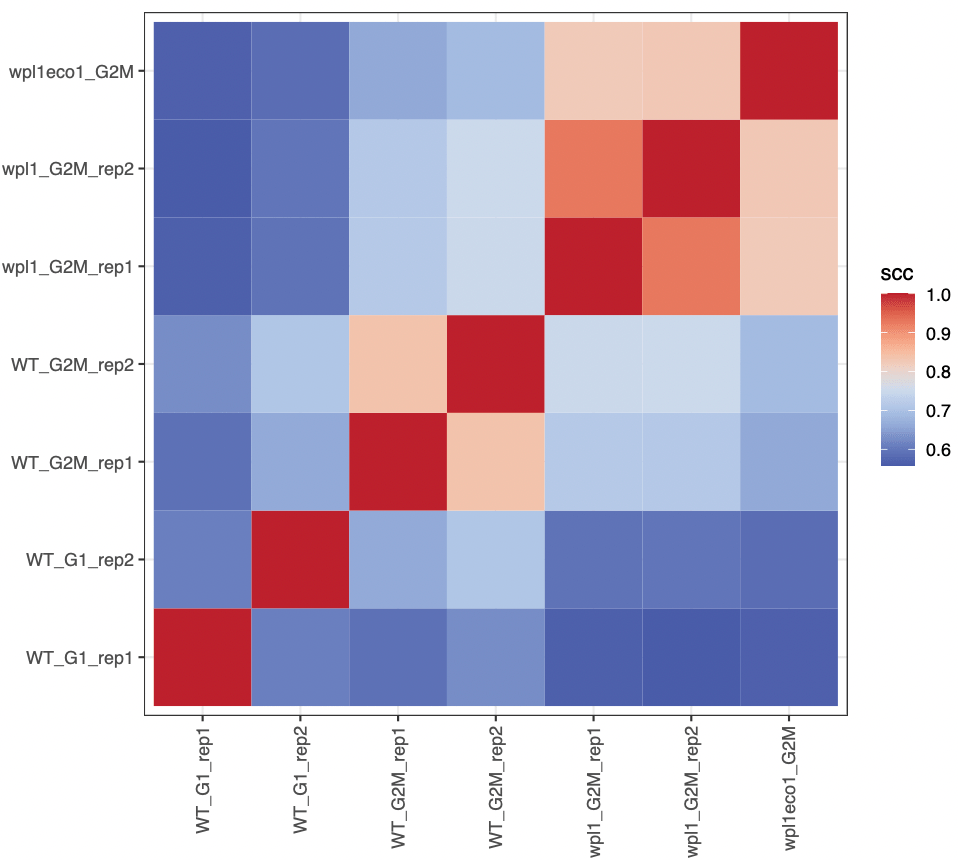We can even iterate over an extra level, to compute stratum-corrected correlation for all chromosomes. Here, we will only compute correlation scores between any sample and `WT_G2M_rep1` sample.

Parallelizing over chromosomes

`BiocParallel::bplapply()` replaces `purrr::map()` here, as it allows parallelization of independent correlation computation runs over multiple CPUs.

``````# Some chromosomes will be ignored as they are too small for this analysis
chrs <- c('II', 'IV', 'V', 'VII', 'VIII', 'IX', 'X', 'XI', 'XIII', 'XIV', 'XVI')
bpparam <- BiocParallel::MulticoreParam(workers = 6, progressbar = TRUE)
df <- BiocParallel::bplapply(chrs, function(CHR) {
mats <- map(hics, ~ .x[CHR] |> interactions() |> gi2cm('count') |> cm2matrix())

map(c(1, 2, 4, 5, 6, 7), function(j) {
data.frame(
chr = CHR,
i = "WT_G2M_rep1",
j = names(mats)[j],
dist = seq(5000, 200000, 1000),
scc = hicrep::get.scc(mats[["WT_G2M_rep1"]], mats[[j]], resol = 1000, h = 2, lbr = 5000, ubr = 200000)
) |> mutate(j = factor(j, names(mats)))
}) |> list_rbind()

}, BPPARAM = bpparam) |> list_rbind()``````

A tiny bit of data wrangling will allow us to plot the mean +/- confidence interval (90%) of stratum-adjusted correlations across the different chromosomes.

``````results <- group_by(df, j, dist) |>
summarize(
mean = Rmisc::CI(scc.corr, ci = 0.90),
CI_up = Rmisc::CI(scc.corr, ci = 0.90),
CI_down = Rmisc::CI(scc.corr, ci = 0.90)
)
ggplot(results, aes(x = dist, y = mean, ymax = CI_up, ymin = CI_down)) +
geom_line(aes(col = j)) +
geom_ribbon(aes(fill = j), alpha = 0.2, col = NA) +
theme_bw() +
labs(x = "Stratum (genomic distance)", y = 'Stratum-corrected correlation')``````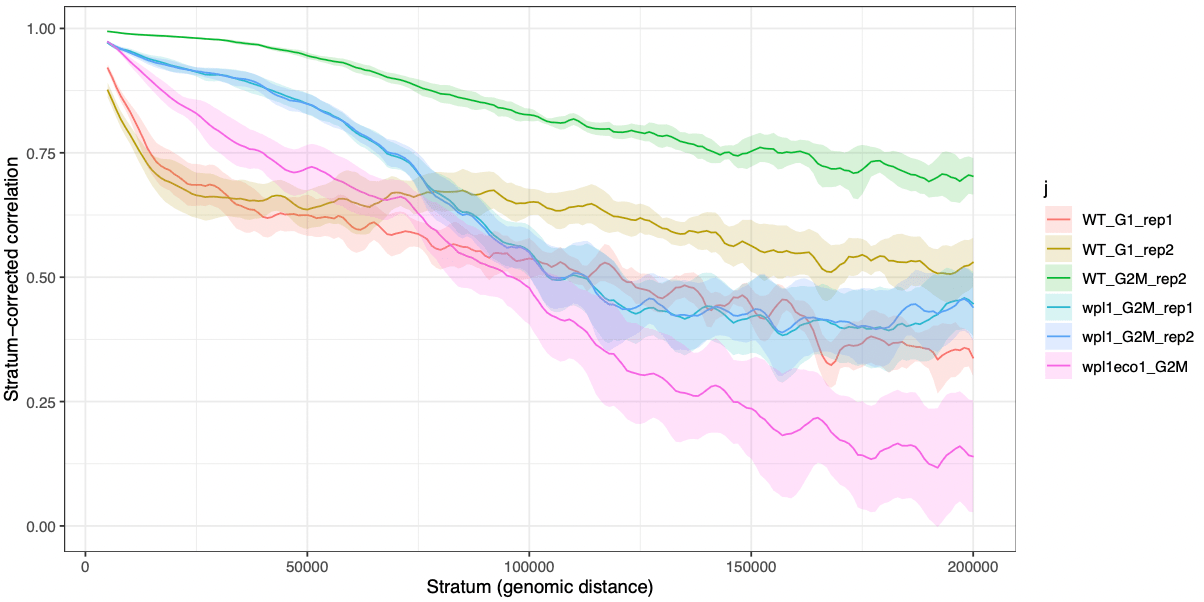## Differential interaction (DI) analysis with `multiHiCcompare`

We will now focus on the chromosome `XI` and identify differentially interacting (DI) loci between WT and wpl1 mutant in G2/M.

To do this, we can use the `multiHiCcompare` package. The required input for the main `make_hicexp()` function is a `list` of raw counts for different samples/replicates, stored in data frames with four columns (`chr`, `start1`, `start2`, `count`).
Although this data structure does not correspond to a standard HiC format, it is easy to manipulate a `HiCExperiment` object to coerce it into such structure.

``````library(multiHiCcompare)
hics_list <- map(hics, ~ .x['XI'] |>
zoom(2000) |>
as.data.frame() |>
select(start1, start2, count) |>
mutate(chr = 1) |>
relocate(chr)
)
mhicc <- make_hicexp(
data_list = hics_list[c(3, 4, 5, 6)],
groups = factor(c(1, 1, 2, 2)
), A.min = 1)``````

The `mhicc` object contains data over the chromosome `XI` binned at 2kb for two pairs of replicates (WT or `wpl1` G2/M HiC, each in duplicates):

• Group1 contains WT data
• Group2 contains `wpl1` data

To identify differential interactions, the actual statistical comparison is performed with the `hic_exactTest()` function.

``````results <- cyclic_loess(mhicc, span = 0.2) |> hic_exactTest()
## |++++++++++++++++++++++++++++++++++++++++++++++++++| 100% elapsed=00s
## |++++++++++++++++++++++++++++++++++++++++++++++++++| 100% elapsed=05s
results
## Hi-C Experiment Object
## 2 experimental groups
## Group 1 has 2 samples
## Group 2 has 2 samples
## Data has been normalized``````

The `results()` output is not very informative as it is. It requires a little bit of reformating to be able to extract valuable insights from it.

``````df <- left_join(results@hic_table, results(results)) |>
mutate(dist = region2 - region1) |>
mutate(group = case_when(
region1 < 430000 & region2 > 450000 ~ 'inter_arms',
region1 >= 430000 & region2 <= 450000 ~ 'at_centro',
TRUE ~ 'arms'
)) |>
filter(group %in% c('arms', 'inter_arms')) |>
mutate(sign = p.value <= 0.05 & abs(logFC) >= 1)
df
## chr region1 region2 D   IF1   IF2   IF3   IF4   logFC  logCPM  p.value    p.adj dist group  sign
##   1       1       1 0  6.16  2.09  7.96  5.43  0.5401 4.81329 5.38e-01 7.94e-01    0  arms FALSE
##   1       1    2001 1 16.38 10.25 12.96 12.16 -0.2257 5.82484 7.00e-01 8.81e-01 2000  arms FALSE
##   1       1    4001 2 41.41 40.72 84.41 45.14  0.5064 7.69885 5.94e-02 2.16e-01 4000  arms FALSE
##   1       1    6001 3 22.26 30.51 73.83 48.48  1.2726 8.10243 6.48e-07 5.83e-05 6000  arms  TRUE
##   1       1    8001 4 26.63 31.20 33.39 25.92  0.0998 7.55207 8.02e-01 9.34e-01 8000  arms FALSE
## ...
ggplot(df, aes(x = logFC, y = -log10(p.value), col = sign)) +
geom_point(size = 0.2) +
theme_bw() +
facet_wrap(~group) +
ylim(c(0, 6)) +
theme(legend.position = 'none') +
scale_color_manual(values = c('grey', 'black'))``````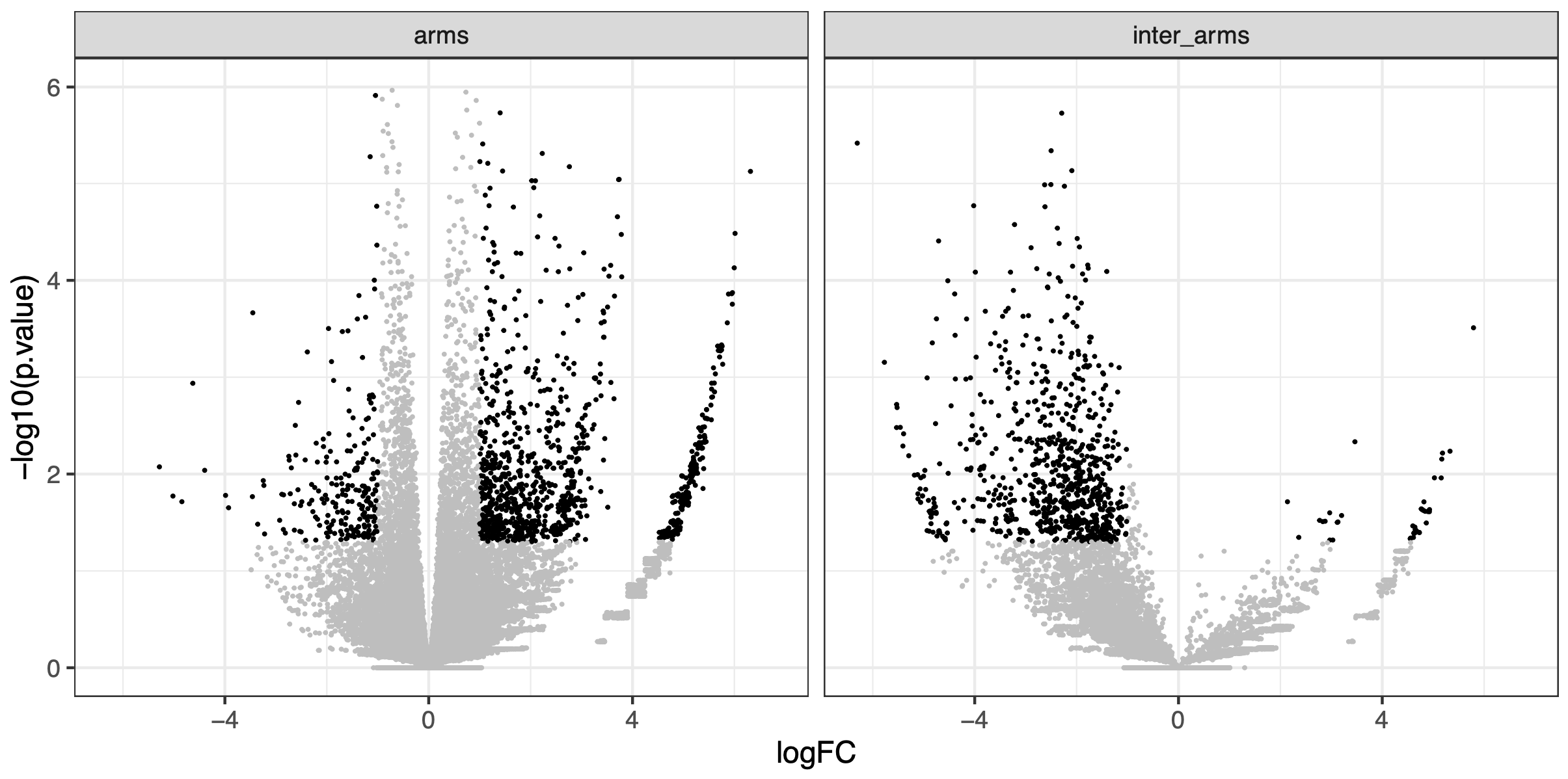In this volcano plot, we can visually appreciate the fold-change of interaction frequency in WT or `wpl1`, for interactions constrained within the chromosome `XI` arms (left) or spanning the chr. `XI` centromere (right). This clearly highlights that interactions within arms are increased in `wpl1` mutant while those spanning the centromere strongly decreased.

One of the strengths of `HiContacts` is that it can be leveraged to visualize any quantification related to genomic interactions as a HiC heatmap, since `plotMatrix` can take a `GInteractions` object with any score saved in `mcols` as input.

``````gis <- rename(df, seqnames1 = chr, start1 = region1, start2 = region2) |>
mutate(
seqnames2 = seqnames1,
end1 = start1 + 1999,
end2 = start2 + 1999
) |>
filter(abs(logFC) >= 1) |>
df2gi()
cowplot::plot_grid(
plotMatrix(merged_replicates[['WT_G2M']], use.scores = 'balanced', limits = c(-3.5, -1), caption = FALSE),
plotMatrix(merged_replicates[['wpl1_G2M']], use.scores = 'balanced', limits = c(-3.5, -1), caption = FALSE),
plotMatrix(gis, use.scores = 'logFC', scale = 'linear', limits = c(-2, 2), cmap = bgrColors()),
align = "hv", axis = 'tblr', nrow = 1
)``````